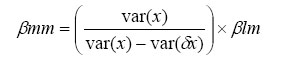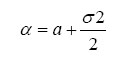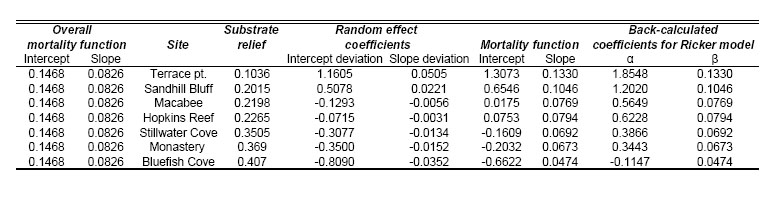### Darren W. Johnson. 2007. Habitat complexity modifies post-settlement mortality and recruitment dynamics of a marine fish. Ecology 88:1716–1725.

Appendix B. Methods for calculating density-independent and density-dependent mortality in the Ricker equation.

Mortality coefficients in the Ricker equation were estimated from the intercept and slope of the estimated mortality function for each site. First, the overall linear model was obtained from the coefficients of the fixed effect (initial density) in a linear, mixed-effects model. Because there was appreciable sampling error in the predictor variable, the coefficients estimated from this model will be biased. To correct for this bias I used a method-of-moments estimator of the slope (Fuller 1987, Carroll and Ruppert 1996). The corrected estimate is calculated by:where var(δx) = measurement error and βlm = slope estimated by the linear model.

In this study measurement error was estimated as the average of the squared standard error of the fish counts. Once the corrected slope was obtained, the intercept was calculated by regressing a line with the corrected slope through the mean values of x and y.

Next, mortality values at each site were calculated from the bias-corrected overall mortality function and the random effects coefficients from the linear, mixed-effects model. The random effects coefficients describe how the estimated values of slope and intercept at each site deviated from the overall mortality function. These values were added to the coefficients of the overall mortality function to yield mortality estimates at each site.

Once slope and intercept parameters were estimated for each site, they were back-calculated to estimate density-independent and density-dependent mortality in the Ricker equation. Because the mortality function assumes a lognormal error structure and because the best-fit linear model passes through modal values, the mortality coefficients were back-calculated such that they represented the arithmetic average of the Ricker function (Hilborn 1985). Values of density-independent mortality (α) were back-calculated as:where σ2 = error variance and a = intercept of the mortality function. Converting to the arithmetic average affects the intercept (density-independent mortality) but not the slope (density-dependent mortality) values (Hilborn 1985, Hilborn and Walters 2001).

A summary of the values used to derive estimates of the mortality coefficients in the Ricker model is given in the following table.LITERATURE CITED

Carroll, R. J., and D. Ruppert. 1996. The use and misuse of orthogonal regression in linear errors-in-variables models. American Statistician 50:1–6.

Fuller, W. A. 1987. Measurement error models. John Wiley and Sons, New York, New York, USA.

Hilborn, R. 1985. Simplified Calculation of Optimum Spawning Stock Size from Ricker's Stock Recruitment Curve. Canadian Jounal of Fisheries and Aquatic Sciences 42:1833–1834.

Hilborn, R., and C. Walters. 2001. Quantitative fisheries stock assessment: Choice, dynamics and uncertainty. Kluwer Academic.

[Back to E088-101]